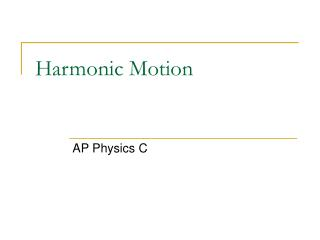DownloadDownload PresentationHarmonic Motion

# Harmonic Motion

Télécharger la présentation## Harmonic Motion

- - - - - - - - - - - - - - - - - - - - - - - - - - - E N D - - - - - - - - - - - - - - - - - - - - - - - - - - -
##### Presentation Transcript

1. Harmonic Motion AP Physics C

2. Springs are like Waves and Circles The amplitude, A, of a wave is the same as the displacement ,x, of a spring. Both are in meters. CREST Equilibrium Line Period, T, is the time for one revolution or in the case of springs the time for ONE COMPLETE oscillation (One crest and trough). Oscillations could also be called vibrations and cycles. In the wave above we have 1.75 cycles or waves or vibrations or oscillations. Trough Ts=sec/cycle. Let’s assume that the wave crosses the equilibrium line in one second intervals. T =3.5 seconds/1.75 cycles. T = 2 sec.

3. Frequency The FREQUENCY of a wave is the inverse of the PERIOD. That means that the frequency is the #cycles per sec. The commonly used unit is HERTZ(HZ).

4. Recall: Hooke’s Law Here is what we want to do: DERIVE AN EXPRESSION THAT DEFINES THE DISPLACEMENT FROM EQUILIBRIUM OF THE SPRING IN TERMS OF TIME. WHAT DOES THIS MEAN? THE SECOND DERIVATIVE OF A FUNCTION THAT IS ADDED TO A CONSTANT TIMES ITSELF IS EQUAL TO ZERO. What kind of function will ALWAYS do this?

5. A SINE FUNCTION!

6. Putting it all together: The bottom line Since all springs exhibit properties of circle motion we can use these expressions to derive the formula for the period of a spring.

7. The simple pendulum If the angle is small, the “radian” value for theta and the sine of the theta in degrees will be equal. mgcosq q mg mgsinq A simple pendulum is one where a mass is located at the end of string. The string’s length represents the radius of a circle and has negligible mass. Once again, using our sine function model we can derive using circular motion equations the formula for the period of a pendulum.

8. The Physical Pendulum A physical pendulum is an oscillating body that rotates according to the location of its center of mass rather than a simple pendulum where all the mass is located at the end of a light string. It is important to understand that “d” is the lever arm distance or the distance from the COM position to the point of rotation. It is also the same “d” in the Parallel Axes theorem.

9. Example A spring is hanging from the ceiling. You know that if you elongate the spring by 3.0 meters, it will take 330 N of force to hold it at that position: The spring is then hung and a 5.0-kg mass is attached. The system is allowed to reach equilibrium; then displaced an additional 1.5 meters and released. Calculate the: Spring Constant 110 N/m Angular frequency 4.7 rad/s

10. Example A spring is hanging from the ceiling. You know that if you elongate the spring by 3.0 meters, it will take 330 N of force to hold it at that position: The spring is then hung and a 5.0-kg mass is attached. The system is allowed to reach equilibrium; then displaced an additional 1.5 meters and released. Calculate the: Amplitude Stated in the question as 1.5 m Frequency and Period 0.75 Hz 1.34 s

11. Example A spring is hanging from the ceiling. You know that if you elongate the spring by 3.0 meters, it will take 330 N of force to hold it at that position: The spring is then hung and a 5.0-kg mass is attached. The system is allowed to reach equilibrium; then displaced an additional 1.5 meters and released. Calculate the: Total Energy 123.75 J Maximum velocity 7.05 m/s

12. Example A spring is hanging from the ceiling. You know that if you elongate the spring by 3.0 meters, it will take 330 N of force to hold it at that position: The spring is then hung and a 5.0-kg mass is attached. The system is allowed to reach equilibrium; then displaced an additional 1.5 meters and released. Calculate the: Position of mass at maximum velocity At the equilibrium position Maximum acceleration of the mass 33.135 m/s/s Position of mass at maximum acceleration At maximum amplitude, 1.5 m